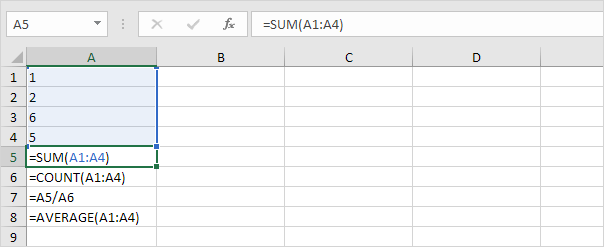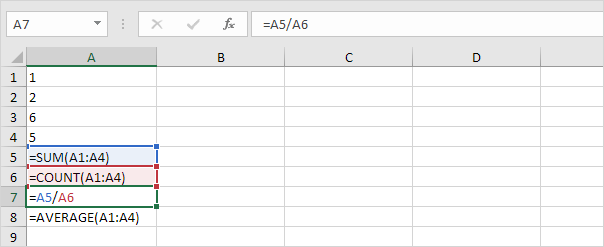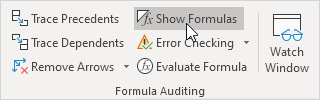# Show Formulas

By default, Excel shows the results of formulas. To show the formulas instead of their results, press CTRL + ` (you can find this key above the tab key).

1. When you select a cell, Excel shows the formula of the cell in the formula bar.2. To display all formulas, in all cells, press CTRL + ` (you can find this key above the tab key).3. Press ↓ twice.Note: as you can see, Excel highlights all cells that are referenced by a formula.

4. To hide all formulas, press CTRL + ` again.

5. If you can't find the grave accent (`) on your keyboard, on the Formulas tab, in the Formula Auditing group, click Show Formulas.6. To hide all formulas, click Show Formulas again.

7. You can also use the FORMULATEXT function in Excel to display formulas in adjacent cells.